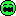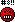Contour Plot

2 replies to this topic

#1vanhoa

vanhoa

Casio Overlord

•• Members
•• 854 posts
• Gender:Male
• Location:Vietnam

• Calculators:
AFX 2.0, CP 300, CP 330, nSpire, TI 89, FX 5800

Posted 22 October 2006 - 11:03 AMI'm finding a methol about Contour Ploting for days but still not found. Help me! May be PAP should know about this.

if 1+1=2 then print "string error" else print "math error" end
if (a+bi)>(c+di) then print "c=d=0; complex error" else "classpad.error: unknow(23): 0x86602" end
if end=end then print "Syntax error" else "Syntax error" end
if contour.possible=true and time.long>1 day then vanhoa.deal=true else vanhoa.badmark=true end

#2PAP

PAP

Casio Overlord

•• Members
•• 681 posts
• Gender:Male
• Location:Somewhere in Europe.
• Interests:Computer Algebra, Numerical Analysis.

• Calculators:
ClassPad 300 (plus an old Casio model, with only a few Kb ram).

Posted 26 October 2006 - 12:10 PMI'm finding a methol about Contour Ploting for days but still not found. Help me! May be PAP should know about this.

if 1+1=2 then print "string error" else print "math error" end
if (a+bi)>(c+di) then print "c=d=0; complex error" else "classpad.error: unknow(23): 0x86602" end
if end=end then print "Syntax error" else "Syntax error" end
if contour.possible=true and time.long>1 day then vanhoa.deal=true else vanhoa.badmark=true end

Contour plots are not easy to implement. To draw a single contour, you need to solve a nonlinear equation for each point. However, you can use a numerical method to solve that nonlinear equation using e.g., built-in function solve, on LNA's functions for this purpose.

#3vanhoa

vanhoa

Casio Overlord

•• Members
•• 854 posts
• Gender:Male
• Location:Vietnam

• Calculators:
AFX 2.0, CP 300, CP 330, nSpire, TI 89, FX 5800

Posted 26 October 2006 - 12:36 PM

Must have a methol I think.

0 user(s) are reading this topic

0 members, 0 guests, 0 anonymous users